# Malus Law

Malus law is crucial if we want to learn or understand the polarization properties of light. The law helps us to study the light intensity relation of polarizer-analyzer. Malus law is named after Étienne-Louis Malus, who in the year 1808 discovered that natural incident light could be polarized when it was reflected by a glass surface. He used calcite crystal for his experiment.

After observing the results, he further put forth a concept that natural light consisted of the s- and p-polarization and that they were perpendicular to each other. Today, this law is used to define the intrinsic connection between optics and electromagnetism as well as demonstrate the transverse nature of electromagnetic waves.

## What is Malus Law?

Malus’ law states that the intensity of plane-polarized light that passes through an analyzer varies as the square of the cosine of the angle between the plane of the polarizer and the transmission axes of the analyzer.

## Malus Law Formula

The law helps us quantitatively verify the nature of polarized light. Let us understand the expression for Malus’ law.

Point 1 – When Unpolarized light is incident on an ideal polarizer the intensity of the transmitted light is exactly half that of the incident unpolarized light no matter how the polarizing axis is oriented.

Point 2 – An ideal polarizing filter passes 100% of incident unpolarized light, that is polarized in the direction of filter’s (Polarizer) Polarizing axis.

From point (1) and point (2) we can assume I = Io cos2 ϕ

The average value of I (< I >):

We know $=<{{I}_{0}}>\,\,\,\,\,<{{\cos }^{2}}\phi > \\ <{{\cos }^{2}}\phi >=1/2$

Which satisfies point (2) mentioned above.

To show point (1), let us consider ϕ = 0

That implies cos2ϕ = 1

I = I0

To determine the direction of polarization we need one polarizer which is known as analyser oriented making an angle (p) with the polarizer.

What happens, when the linearly polarized light emerging from a polarizer passes through a second polarizer (analyser) in general the polarizing axis of the second polarizer (analyser) makes an angle (d) with the polarizing axis of the first polarizer.

Since the intensity of an electromagnetic wave is proportional to the square of the amplitude of the wave, the ratio of transmitted to incident amplitude is cos ϕ, so the ratio transmitted to incident intensity is cos2ϕ

$\begin{matrix} \cos \phi =\frac{A}{{{A}_{\max }}}=\frac{Transmitted\,amplitude}{incident\,amplitude} \\ \cos \phi =\frac{A}{A\max }=\sqrt{\frac{I}{{{{I}}_{max}}}} \\ then\,\frac{I}{{I}{}_{max}}={{\cos }^{2}}\phi \\ I={{{I}}_{max\,}}\cos {}_{2}\phi \\ \end{matrix}$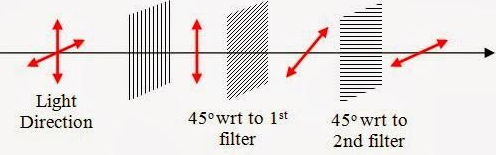## Solved Problems and Questions

1. Which wave can be polarized?

Solution:

Lightwave gets polarised. The phenomenon of polarization takes place only in the transverse nature of waves. So sound waves cannot be polarised.

2. What is the difference between unpolarized light and plane-polarized light?

Solution:

 Unpolarised light Plane polarised light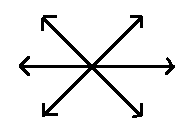The orientation of electric field vectors $\left( \overrightarrow{E} \right)$ will be in all possible directions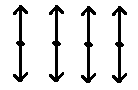The orientation of Electric field vectors $\left( \overrightarrow{E} \right)$ will be in a direction on a plane perpendicular to the direction of prorogation of light.

3. An Unpolarised light with intensity (I) is passing through a polarizer. What happens to the intensity of incident light?

Solution:

An unpolarized light of intensity (I) passes through a polariser, outcoming light intensity becomes half of its initial value$\left( \frac{I}{2} \right)$.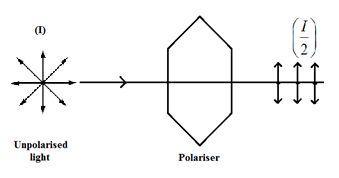4. An unpolarised light passes through two successive polaroids (P1 and P2) the polaroid P1 makes angle θ with the axis of the polaroid P2. Find out the intensity final out coming light? And if θ is varied from 0 to 27. Plot the intensity variation graph?

Solution:

An unpolarised light passes through two successive polaroids.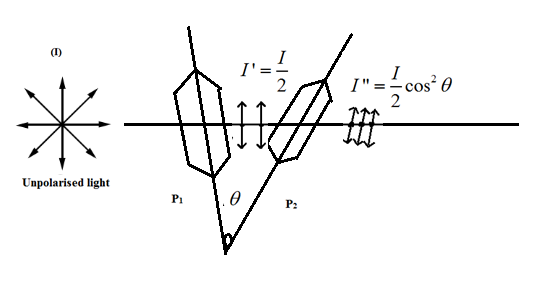We know, an unpolarized light passes through a polaroid its intensity becomes half of its initial intensity. If it passes again through any other polaroids, then its intensity given by Malus law is,

$I=\frac{I}{2}{{\cos }^{2}}\theta$

θ – is the angle between the axis of the polaroids intensity variation with respect to 0 to 2π. It is nothing (cos2 θ) curve.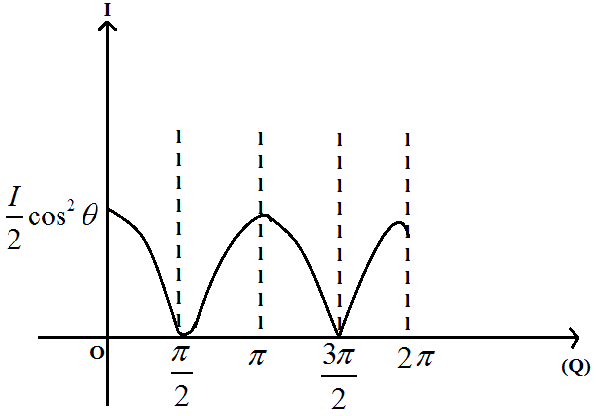5. How an Unpolarised light of intensity (I0) get plane-polarized when passing through a polaroid?

(i) An Unpolarised light of intensity (I0) passes through two successive polaroids (P1 and P2) and corresponding intensities of light coming out from them is (I1 and I2) clearly distinguish the difference between (I1) and (I2).

(ii) What is the necessary condition to get maximum intensity after passing through two successive polaroids?

Solution:

As we know from Malus’ law, an unpolarized light passes through a polaroid, electric field $\left( \overrightarrow{E} \right)$ vector of unpolarised light get polarised along a direction in a plane perpendicular to the direction of propagation of light. The intensity of the plane polarised light becomes half its incident light intensity $\left( \frac{I}{2} \right).$ . Then this plane polarised light passes through another polaroid called analyser, the outcoming light, intensity as a function of cosine square angle between the plane of polariser and analyser.

$I\propto {{\cos }^{2}}\theta$

Where θ – is the angle between the plane of the polarizer and analyser.

$I={{I}_{0}}{{\cos }^{2}}\theta$

The necessary condition for intensity maximum,

$\cos \theta =\pm 1$

θ should be $\theta =0\,\,\left( or \right)\,\,\,\pi$

The angle (θ) between the plane of transmission of polariser and analyser must be zero (or) the polarizer and analyser must be parallel to each other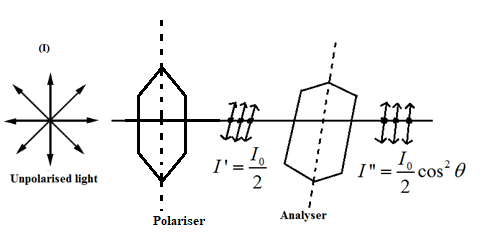When $\cos \theta =\pm 1$

Then I = I0

When $\cos \theta =0$

The I = 0

Intensity coming out from polaroid P1 and P2 (I1 and I2)

From Malus law

$I={{I}_{0}}{{\cos }^{2}}\theta$

We know the average value of ${{\cos }^{2}}\theta \simeq \frac{1}{2}$ $<{{\cos }^{2}}\theta >=\frac{1}{2}$

When (I0) intensity of unpolarised light passes through a polaroid (P1) its intensity becomes (I1), an unpolarized light have electric field vector in all possible directions, when they pass through a polaroid they get polarised along a direction, from Malus law $I={{I}_{0}}{{\cos }^{2}}\theta$ ${{I}_{1}}=\frac{{{I}_{0}}}{2}$

Then the same unpolarised light of intensity (I0) after passing through a polaroid its intensity becomes (I1) and this (I1) passes through second polaroid (Analyser) then its intensity becomes (I2).

${{I}_{2}}={{I}_{1}}{{\cos }^{2}}\theta$

It is a function of the square of the cosine angle (θ) between the arms of polarisation of polaroid and analyser.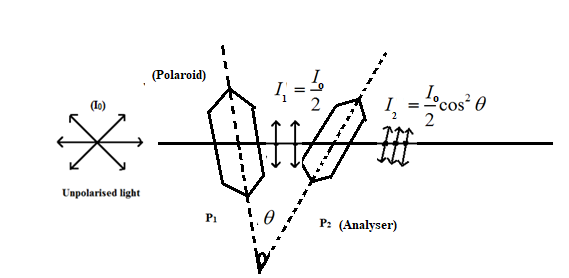6. An Unpolarised light of intensity (I0) passes through three successive polaroids P1, P2 and P3. Polarisation axes of P1 make an angle 60° with P2 and P2 makes angle 30° with P3. The corresponding intensity outcomes from P1, P2 and P3 (I1, I2 and I3) is what?

Solution:

${{I}_{1}}=\frac{{{I}_{0}}}{2}$ (corresponding to P1)

${{I}_{2}}={{I}_{1}}{{\cos }^{2}}\theta$ (corresponding to P2)

$=\frac{{{I}_{0}}}{2}\left( {{\cos }^{2}}60{}^\circ \right)$ $=\frac{{{I}_{0}}}{2}{{\left( \frac{1}{2} \right)}^{2}}=\frac{{{I}_{0}}}{8}$ ${{I}_{3}}={{I}_{2}}{{\cos }^{2}}\theta$ (corresponding to P3)

$=\frac{{{I}_{0}}}{8}{{\cos }^{2}}\left( 30{}^\circ \right)$ $=\frac{{{I}_{0}}}{8}{{\left( \frac{\sqrt{3}}{2} \right)}^{2}}=\frac{3{{I}_{0}}}{32}$